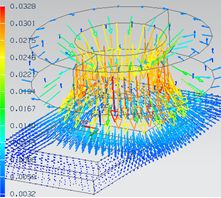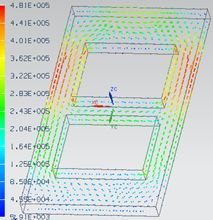# Magnetodynamics - Frequency-domainThe magneto-dynamic solution in frequency domain describes the distribution of magnetic fields and eddy currents due to time harmonic excitation loads.

We use a quasi stationary approximation. The assumptions are applicable in cases of dimensions << wavelength. Examples are motors, transformers and frequencies from 0 Hz to a few 100 kHz.

## Features

• 2D, 3D or axisymmetric Solution
• coupled with Thermal solution possible
• Outputs Plot:
• Magnetic Fluxdensity, Magnetic Fieldstrength, Current Density, Eddy Current Losses Density, Hysteresis-, Eddy-, Excess Loss Density - steinmetz, Magnetic Potential (a-Pot).
• Outputs Table:
• RotorBand Torque - stresstensor, Magnetic Potential on Conductors - Fluxlinkage, Voltage on Coils, Voltage on Circuits, Electrode Voltage, Electrode Current, Current on Circuits, Power on Circuits, Eddy Current Losses, Hysteresis-, Eddy-, Excess Losses, Ohm Resistance, Coil Inductivity, Phase Shift.

## Theory and Basics

### Formulations

The basis equations:
(1)        rot h = j
(2)        rot e = -δt b
(3)        div b = 0

Constitutive relations:
(4)        b = µ h
(5)        j = σ e

#### a-Formulation

The following a-formulation is used for 2D-Magnetodynamics.

Magnetic vectorpotential a:
(6)        b = rot a
(7)        e = -δt a

Magnetodynamic  weak  a-formulation:
(8)            ( µ-1 rot a, rot a’ )Ω
+ (-µ-1 bs, rot a’ ) Ω

+ (- ja’ ) ΩC
+ (σ δt aa’ ) Ωc

= 0, for all a’ element of Ω

#### a-v-Formulation

The following a-v-formulation is used for 3D-Magnetodynamics.

Magnetic vectorpotential a, electric scalar potential v:
(9)         b = rot a
(10)       e = -δt – grad v

Magnetodynamic  weak  a-v-formulation:
(11)           (µ-1 rot a, rot a’ )Ω
+ (σ δt aa’ ) Ωc
+ (σ grad v, a’ ) Ωc
+ (σ δt a, grad v’ ) Ωc
+ (σ grad v, v’ ) Ωc

= 0

### Basic Example: Team Problem 3: The Bath Plate

The problem 3 of the TEAM (Testing Electromagnetic Analysis Methods) is one of the examples for testing eddy current codes. A conductive plate with two holes is placed under a coil. The coil is driven by alternating current of 50 Hz and 1260 ampere turns. The goal is to analyze for the magnetic fluxdensity along a line that goes slightly over the plate.The Mesh is shown in the following picture.#### Results: Fluxdensity and  Induced Current

The magnetic fluxdensity as contourplotThe magnetics fluxdensity as vectorplotThe induced current density as contourpolotThe induced current density as vectorplot